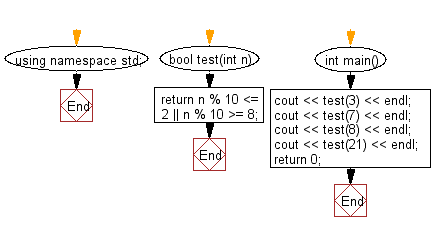﻿ C++ : Check if a number is within 2 of a multiple of 10# C++ Exercises: Check if a given number is within 2 of a multiple of 10

## C++ Basic Algorithm: Exercise-41 with Solution

Write a C++ program to check if a given number is within 2 of a multiple of 10.

Sample Solution:

C++ Code :

``````#include <iostream>

using namespace std;

bool test(int n)
{
return n % 10 <= 2 || n % 10 >= 8;
}

int main()
{
cout << test(3) << endl;
cout << test(7) << endl;
cout << test(8) << endl;
cout << test(21) << endl;
return 0;
}
``````

Sample Output:

```0
0
1
1
```

Flowchart:C++ Code Editor:

Contribute your code and comments through Disqus.

What is the difficulty level of this exercise?

﻿